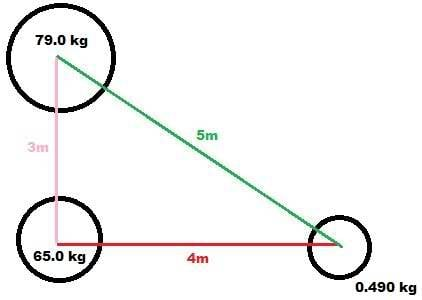# What is the magnitude of the net gravitational force

Gravitation Force (Solved, thanks)

## Homework Statement

A sphere with mass of 65kg and center at origin. Another sphere with mass 79kg at point (0,3), also centered at origin.

A.) What is the magnitude of the net gravitational force due to these objects on a third uniform sphere with mass 0.490 kg placed at the point (4,0)

## Homework Equations

$$F_{g} = \frac{GMm}{r^{2}}$$
$$G = 6.67(10^{-11})$$

## The Attempt at a Solution

I made this image to help:Force from the 79kg sphere:
$$FG_{1}=\frac{G(.490)(79)}{5^{2}}$$

Force from the 65kg sphere
$$FG_{2}=\frac{G(.490)(65)}{4^{2}}$$

So the net force on the .490 kg sphere is given by:
$$\sqrt{(FG_{2} + FG_{1}cos(tan^{-1}(\frac{3}{4})))^{2}+(FG_{1}sin(tan^{-1}(\frac{3}{4})))^{2}}$$

I get the net force on the 0.490 kg sphere to be 3.48 N. Which is incorrect? I don't know what is wrong.

Last edited:

ehild
Homework Helper
What did you use for G?

You can find the cosine and sine of the angle at the 0.49 kg mass directly from the right triangle.

ehild

What did you use for G?

You can find the cosine and sine of the angle at the 0.49 kg mass directly from the right triangle.

ehild

I used 6.67 * 10^-11 for G.

What do you mean about the cos/sin ? arctan(3/4) is the angle near the .490kg sphere, so the equation should hold?

ehild
Homework Helper
I used 6.67 * 10^-11 for G.

What do you mean about the cos/sin ? arctan(3/4) is the angle near the .490kg sphere, so the equation should hold?

The sine of the angle is opposite/hypotenuse=3/5. The cosine is adjacent/hypotenuse=4/5. Is not that simpler than taking the cosine and sine of arctan(3/4)? It is not wrong, just strange.

Check your calculation. I think you forgot the 10^-11.

ehild

You are correct. I don't know why I was thinking that I needed to determine the angle via inverse trig.

I did the computations over and somehow it came out correct this time.
It was 2.24*10^5 N. I must have missed something somewhere.

Thanks

Now I am working through this part:

Where, other than infinitely far away, could the third sphere be placed such that the net gravitational force acting on it from the other two spheres is equal to zero?

But I'm still working it out.

I am thinking that:
$$\frac{G(.409)(79)}{d^{2}} = \frac{G(.409)(65)}{(3-d)^{2}}$$
$$\frac{(79)}{d^{2}} = \frac{(65)}{(3-d)^{2}}$$
$$9-6d+d^{2}=\frac{65}{79}d^}{2}$$

so basically the third sphere needs to be at the point (0,1.43) ?

Edit: Yeah thats all correct!

Thanks for the help.

Last edited: